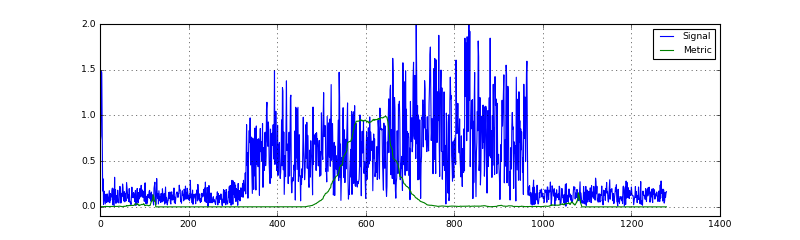# Using Schmidl&Cox synchronization algorithm for obtaining the symbol start¶

In the previous notebook, we have implemented straight-forward OFDM modulation and demodulation. There, we have seen, that the information about where the symbol starts (and hence, where to put the FFT window) is crucial for a successful demodulation. For the simulated channel, we could calculate the delay and hence get a decent constellation diagram. However, for the real audio channel, the delay and hence the timing offset occurs random and hence at the receiver side we need to estimate the symbol timing from the received signal.

A common methodology used for OFDM has been published by Schmidl & Cox in 1997. In this notebook, we will use their proposal to estimate the start of each OFDM symbol. A detailed treatment of the algorithm is available e.g. in a DSPIllustrations article. Instead of going into the mathematical details here, we will focus on the implementation of the algorithm in the following.

First, we need to import basic building blocks from the communications library:

In :
from audioComms.components import Component,TX, Environment, Recorder
from audioComms.utils import StatefulFilter, get_filter
from audioComms.plotting import PlotSpectrum, PlotConstellation, PlotBlock, PlotWaveform
from audioComms.channels import AudioChannel, SimulatedChannel, IdentityChannelEffect, SimulatedChannelEffect, NoiseChannelEffect, InitialLossChannelEffect, ChainedChannelEffects


In addition to the common imports from above, we import basic functions for OFDM systems, that match the implementations from the previous notebook:

In :
from audioComms.ofdm import OFDM, random_qam, ofdm_modulate, MostBasicOFDMReceiver


## Effect of asynchronicity¶

Before we proceed on using the Schmidl&Cox algorithm, let us look a bit further into what happens when an OFDM symbol is not accurately synchronized. To do this, we create a basic transmitter that repeatedly transmits the signal passed in into the constructor:

In :
# Source code has been redacted in online version
# Omitting 7 lines of source code


In addition, let us use the MostBasicOFDMReceiver from the previous notebook to illustrate how the received frames look like and where the FFT window is put. As a specifity, the receiver takes a correctly synchronized OFDM symbol as a reference to display it later on.

In :
class IllustrativeOFDMReceiver(MostBasicOFDMReceiver):
def __init__(self, environment, ofdm, correctSymbol):
super().__init__(environment, ofdm)
self._correctSymbol = correctSymbol       # record the genie-aided, correctly synchronized OFDM symbol

def _processOFDMSymbol(self, symbol):
# called for each extracted OFDM symbol
assert len(symbol) == self._symbolLength

# calculate the indices, where the FFT window would be positioned: after the CP
rxWindow = np.zeros(len(symbol))
rxWindow[self._ofdm.CP:] = 1

# forward the received symbol, FFT window and correct (i.e. reference) symbol to a debug stream
self._send((rxWindow,
abs(symbol),
abs(self._correctSymbol),
dict(legend=("FFT Window", "RX symbol", "Correct Timing"))),
stream='symbol')   # send the time-domain signal to a debug stream

# attempt to OFDM demodulate the symbol and forward the constellation symbols
payload = symbol[self._ofdm.CP:]           # remove CP
X = np.fft.fft(payload)                    # simply do FFT, no equalization yet.
self._send(X)



Let us use above receiver to illustrate the effect of a miss in the correct symbol start. To be very explicit, we create a specific OFDM symbol which equals a Dirac impulse: $$x[n]=\delta[n].$$ As we know, we have $$X[k]=\text{DFT}_K\{x[n]\},$$ i.e. the constellations symbols that belong to $x[n]=\delta[n]$ are given by $$X[k]=\text{DFT}_K\{\delta[n]\}=1,$$ i.e. as constellation symbols we expect all constellation symbols to be located at 1+0j (*note that this is actually not a valid QPSK point; however for the present illustration this is perfectly fine).

Let us write a simple connection chain for illustrations purposes:

In :
# Source code has been redacted in online version
# Omitting 31 lines of source code


Let's see what happens if we run this with no delay:

In :
showEffectOfAsynchronicity(0)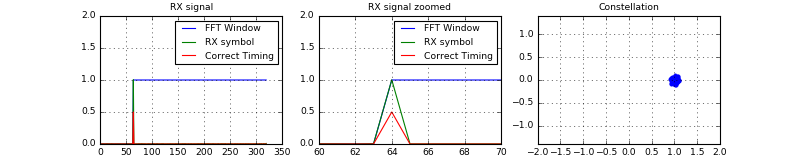OK, the received signal (i.e. the Dirac pulse) is correctly timed and the FFT window starts exactly at the impulse. Hence, the constellation are located around the expected $1$ with just a little bit of noise on top.

Now, let's do some more analysis and let the symbol be slightly (i.e. one sample) offset in time. First, we delay by exactly one OFDM symbol (i.e. K+CP samples), which should end up with a correct timing again. Then, we shift this timing by one to the front and to the back:

In :
display(HTML("<h4>Correct timing<h4>")); showEffectOfAsynchronicity(256+64)
display(HTML("<h4>One sample too early<h4>")); showEffectOfAsynchronicity(256+64-1)
display(HTML("<h4>One sample too late<h4>")); showEffectOfAsynchronicity(256+64+1)


#### Correct timing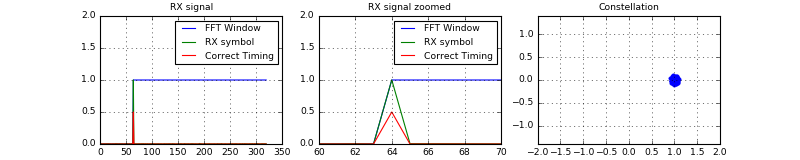#### One sample too early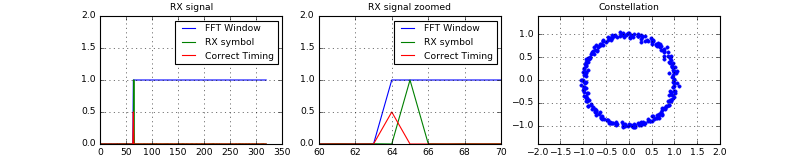#### One sample too late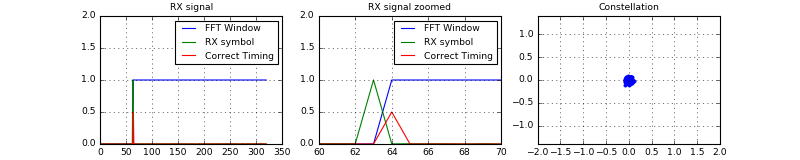Uh, just one sample offset completes messes up the constellation diagram! If we start the FFT window too early (second row), the obtained constellation becomes a circle. If we sample too late (third row) we do lose the constellation completely?

#### Second row: one sample too early:¶

In our special case of sending a Dirac impulse, we can actually directly calculate the expeceted constellation when we start the FFT window one sample too early. In this case, the received symbol equals $x[n]=\delta[n-1]$ and hence $$X[k]=\text{DFT}_K\{\delta[n-1]\}=\exp(j2\pi k/K)$$ which equals a circle.

#### Third row: one sample too late:¶

In our special case of sending a Dirac impulse, we completely miss the Dirac peak, and hence the FFT window only contains zeros. However, in reality, we do not transmit Diract pulses and hence the time-domain of the OFDM symbol would not be completely lost. Still, if we sample the signal too late, we miss the first few samples of the symbol. In constrast to sampling too early, here the CP cannot recover this loss.

In summary, sampling the OFDM symbol at a wrong point in time messes up the constellation diagram (if we sample too early) or even loses samples (sample too late). As the time-shift introduced by sampling too early can be recovered by a decent channel estimation (see next notebook), it is advisable to rather sample too early than too late.

In the following, we will exploit the Schmidl&Cox metric to estimate the symbol start:

## The Schmidl&Cox Metric¶

In short, the Schmidl&Cox metric is based on an OFDM symbol $x[n]$ that contains two repetitive parts of length $K/2$, i.e. $x[n]=x[n+K/2]$. Let us create such a symbol below. To achieve a repetition, we leverage a DFT property: Upsampling in frequency domain corresponds to repetition in time domain. Hence, if we create frequency domain data with a zero at every second frequency, the corresponding time-domain signal consists of two repetitions:

In :
def createSchmidlCoxPreamble(ofdm):
qam = random_qam(ofdm)
qam[::2] = 0
ofdm_symbol = ofdm_modulate(ofdm, qam)
return ofdm_symbol


Let us verify this:

In :
ofdm = OFDM(K=256, Kon=200, CP=64)
preamble = createSchmidlCoxPreamble(ofdm)
preamble_noCP = preamble[ofdm.CP:]
plt.figure(figsize=(8,2))
plt.plot(abs(preamble_noCP))
plt.grid(True); plt.xlim((0, len(preamble_noCP)));
plt.axvline(ofdm.K//2, color='red'); plt.title('The Schmidl-Cox Synchronization Sequence');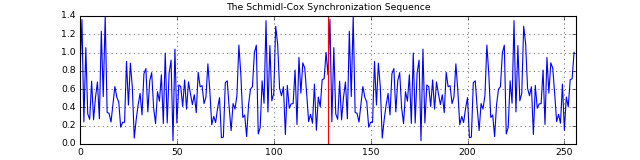Right, the symbol consists of two repeated parts. We have center the middle by the red line.

Now, for a received signal $r[n]$ the Schmidl&Cox metric is defined as follows. First, we have the submetric $P[n]$ given by

$$P[n] = \sum_{m=0}^{L-1}r^*[n+m]r[n+m+L]$$

where $L=K/2$ is the length of one repetitive part. This metric is non-causal (as for P[n] we need access to the value of $r$ at times $n+K$ for example. In the DSPIllustrations.com article on Schmidl&Cox synchronization we have transformed this non-causal filter to a causal one. In essence, we define the signal $\bar{v}[n]=r[n-L]^*r[n]$ and then define the causal metric recursively as $$\bar{P}[n+1]=\bar{P}[n]+\bar{v}[n]-\bar{v}[n-L]=\sum_{m=0}^{L-1}\bar{v}[n-m].$$ This shows us, that the metric $\bar{P}$ can be calculated by applying a linear filter with rectangular impulse response to the signal $\bar{v}[n]$. In addition, to calculate $\bar{v}[n]=r[n-L]^*r[n]$ we need to delay $r[n]$ by $L$ samples, which can be accomplished by another linear filter.

The second submetric $\bar{R}[n]$ is given by

$$\bar{R}[n]=\sum_{m=0}^{L-1}|r[n-m]|^2$$

which again can be calculated by applying a rectangular filter to the signal $|r[n]|^2$.

First, let's create these two filters and look at their impulse response:

In :
# Source code has been redacted in online version
# Omitting 12 lines of source code

In :
# Source code has been redacted in online version
# Omitting 15 lines of source code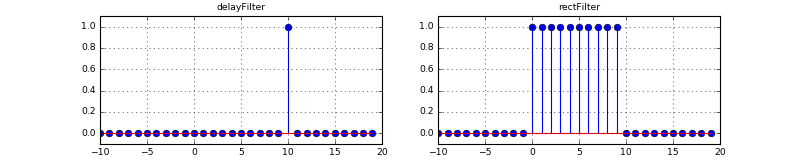As we wanted, we have created both a rectangular filter that sums the last $L$ samples and a filter which delays a signal by $L$ samples, where $L=10$ in the example above.

The final metric in its causal version for the Schmidl&Cox synchronization technique is then given by

$$\bar{M}(n)=\frac{|\bar{P}[n]|^2}{|\bar{R}[n]|^2}.$$

In the following class SchmidlCoxMetric, we calculate the metric according the equations above:

In :
# Source code has been redacted in online version
# Omitting 36 lines of source code


Let us try out this component in a transmission in the bandpass frequencies, such that we can also run it with the real audio channel. First, we import the Passband channel class, which combines upconversion, physical transmission and downconversion:

In :
from audioComms.passband import PassbandChannel


Now, we define a function to connect the different components:

In :
def runSchmidlCoxMetric(channelFunc):
ofdm = OFDM(K=256, Kon=200, CP=64)
preamble = createSchmidlCoxPreamble(ofdm)

env = Environment(samplerate=44100)
Fc = 10000  # carrier frequency
B = 441     # baseband sampling rate or bandwidth

tx = AlwaysTheSameSignalTransmitter(env, preamble)   # repeatedly transmit only the preamble
channel = PassbandChannel(env, channelFunc=channelFunc, Fc=Fc, B=B)

scMetric = SchmidlCoxMetric(env, ofdm, minEnergy=1)   # calculate the SC metric ...
showWave = PlotWaveform(env, figsize=(10,3), ylim=(-0.1, 2), numLines=2,   # ... and plot it
integerXaxis=True, windowDuration=4*(ofdm.K+ofdm.CP))

# set up the connections between the blocks
tx.transmitsTo(channel)
channel.transmitsTo(scMetric)
scMetric.transmitsTo(showWave)

env.run()


Let us first try out with an ideal SimulatedChannel:

In :
runSchmidlCoxMetric(SimulatedChannel)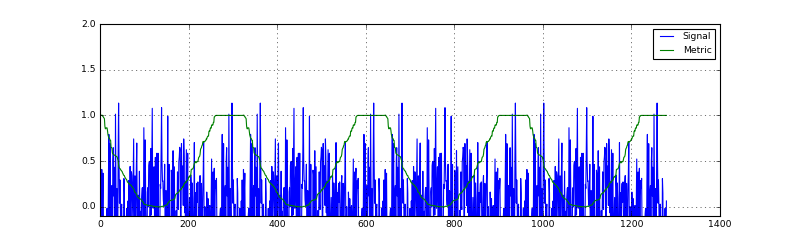Stop received from external


Great! We have a periodic repetition of the SC metric. This indicates, that the metric is working and that it can detect the preamble. Unfortunately, for now we cannot really see how the metric relates to the actual signal. We will resolve this in a minute. For now, let us first ensure that also over the audio channel we can obtain a reasonable metric:

In :
runSchmidlCoxMetric(AudioChannel)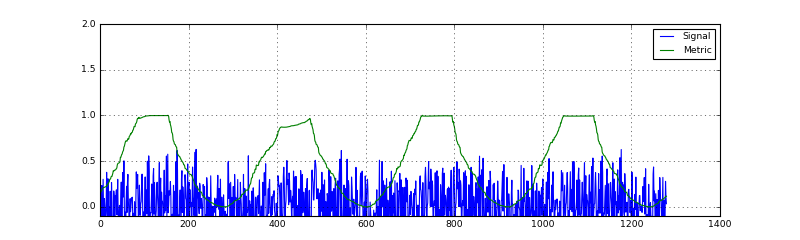Stop received from external


Yes! The metric that is received from the audio channel shows a similar behaviour as in the simulation! This means, we really have a chance to synchronize and detect our OFDM signals over the acoustic channel. Hint: Try changing the baseband bandwidth B and see what happens to the metric. Make sure that 44100 % B == 0.

Note: If you cannot see any metric output, try decreasing the minEnergy parameter of the SchmidlCoxMetric call or increase the microphone volume.

## Extracting the Symbol start from the Schmidl-Cox Metric¶

In the previous section we have seen that also over the acoustic channel, we get a reasonable output of the metric. However, the metric alone wont help us in synchronization. We also need to know, how the metric shape relates the position of the OFDM symbol starts.

To analyze this, we first set up another simulation, where we record the metric and the signal such that we can process it offline. In addition, we slightly modify our signal such that it looks as follows:

In :
%%tikz -l positioning -s 800,400
\draw [->] (-1,0) -- (19,0) node [below,right] {$t$};
\foreach \x in {0,1,2} {
\draw [fill=red!50!white] (6*\x,0) rectangle +(2,1) node [midway] {Preamble};
\draw [fill=blue!50!white](6*\x+2,0) rectangle +(2,1) node [midway] {Noise};
\draw [fill=brown] (6*\x+4,0) rectangle +(2,1) node [midway] {Zero};
}Accordingly, we can be sure that the first sample after the zero signal is the first sample of the preamble (i.e. the S&C synchronization sequence), and hence we can infer the entire timing of the signal.

Here's the function to record the metric:

In :
# Source code has been redacted in online version
# Omitting 32 lines of source code


Let's run this and see the output:

In :
ofdm = OFDM(K=256, Kon=200, CP=64)
np.random.seed(2)  # fix the random generator to create reproducable results
preamble = createSchmidlCoxPreamble(ofdm)
recordedSamples = recordSchmidlCoxMetric(lambda env: SimulatedChannel(env, NoiseChannelEffect(0.00)), duration=3, preamble=preamble)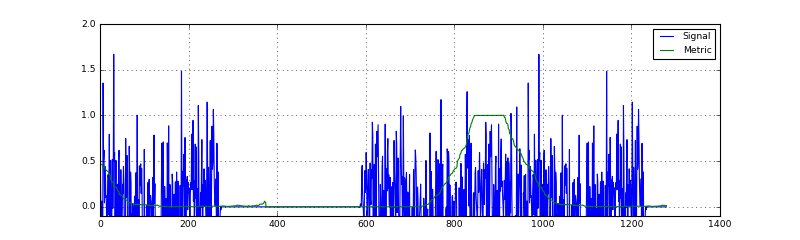OK, we see the common shape of the SC metric, but in addition we see smaller peaks. Let's look at the recorded signals to find out what they are, and how the timing is in general:

In :
signal = recordedSamples
metric = recordedSamples
plt.figure(figsize=(12,3))
plt.subplot(121)
plt.plot(signal.real);
plt.plot(metric.real, 'r');
plt.ylim((-0.1,2))
plt.grid(True);

plt.subplot(122)
plt.plot(signal.real);
plt.plot(metric.real, 'r');
plt.xlim((300, 350)); plt.grid(True)
plt.axvline(326, color='green');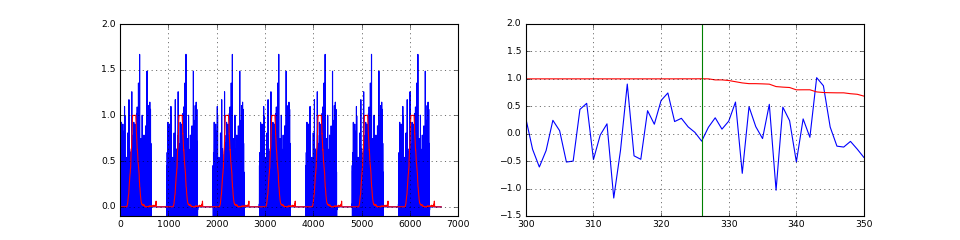OK, the small peaks occur within the region where the signal is zero. We remember, we have $R[n]$ being the signal energy of the $L$ samples before $n$. Moreover, $M[n]=P[n]/R[n]$. Hence, looking at the metric, we infer that the signal energy $R$ goes down to zero and hence we significantly amplify the metric in these area. That's why we have the extra check regarding minEnergy in the metric calculation. However, this can only mitigate the problem a little bit and a small artifact remains. However, as the peak is much smaller than the actual metric we wont care about it.

Let's consider more what the useful metric is doing. From the previous notebook, we remember that the passband channel introduces a delay of 6 baseband samples. As we start the signal with a preamble and the preamble has a length of $K+N_{CP}=256+64=320$ samples we know that the preamble ends at sample $6+256+64=320$. We have indicated this sample with the red line in the rightmost figure, where we have zoomed into the signal to see the metric. Apparently, when the metric leaves the plateau (which is caused by the CP and has the length of the CP), the preamble ends. We can use this information to calculate the start of the preamble.

What remains is the problem to detect the point when the metric leaves the plateau. To find this point, we employ a straight-forward algorithm, which can be described as follows:

1. Compare the metric against a fixed threshold.
2. Detect each region, where the metric is above this threshold.
3. Calculate the center of this region. Assuming that the metric rises and falls in a symmetric manner, the center of the region is the center of the plateau.
4. From the center of the region advance by $N_{CP}/2$ samples to find the end of the plateau.

This algorithm is implemented below:

In :
def detectOFDMSymbols(ofdm, metric, signal):
above = (metric.real > 0.5).astype(int)   # Perform thresholding
edges = np.diff(above)               # Detect points where the thresholded signal changes
edgeInds = np.flatnonzero(edges)
edgeVals = edges[edgeInds]

centers = []

lastRise = -1
for i, v in zip(edgeInds, edgeVals):   # iterate over all changes
# check for falling edge
if v == -1 and lastRise != -1: # we are at the end of a metric peak
center = (lastRise + i) // 2   # the center is in the center between the current edge and the previous rising one
centers.append(center)

# check for rising edge
if v == 1:
lastRise = i  # remember position of rising edge

centers = np.array(centers)
CP = 64
preambleEnds = centers + CP//2-3   # Obtain the end of the preamble by adding half of the CP plus some correct factor

symbols = []
symbolLen = ofdm.K
# for each detected preamble, extract it from the signal and store it
for end in preambleEnds:
symbols.append(signal[end-symbolLen:end])

# plot the different steps
plt.figure(figsize=(10,3))
plt.plot(metric.real, label='Metric')
plt.plot(above, label='Metric above threshold')
plt.plot(edges, label='Edge')
plt.stem(preambleEnds, np.ones(len(preambleEnds)), label='Detected preamble ends')
for c in centers:
plt.axvline(c, ls='--')
plt.legend()

# return the extracted symbols
return symbols
symbols = detectOFDMSymbols(OFDM(K=256, Kon=200, CP=64), metric, signal)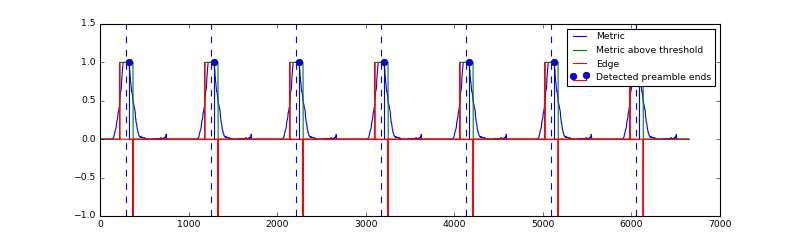OK, the proposed algorithm detects the center of the preamble peaks and subsequently calculates the end of the plateau and hence of the preamble. Let us see, if the extracted symbols match the expected preamble and if we can obtain a constellation diagram from them:

In :
plt.figure(figsize=(10,3))
plt.subplot(121)
# plot the extracted symbols and the expected timing
plt.plot(abs(preamble[ofdm.CP:]), lw=2);
plt.plot(abs(np.array(symbols).T))
plt.grid(True)

# attempt demodulation and show the resulting constellation diagram
X = [np.fft.fft(s) for s in symbols]
plt.subplot(122); plt.axis('equal')
for x in X:
plt.plot(x.real, x.imag, 'o')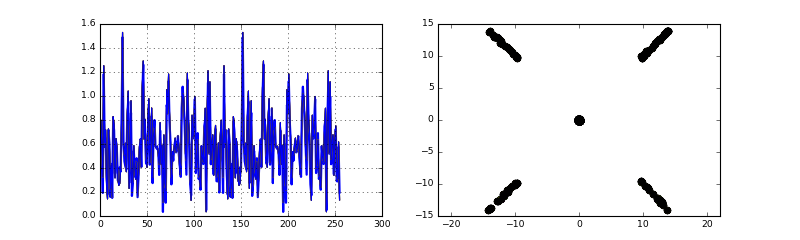Yes! The extracted symbols exactly match the correctly timed signal. Moreover, we can obtain a decent constellation diagram. But, have you observed the "correction factor" in the the line preambleEnds = centers + CP//2-3? This factor was added here to make the timing exactly correct. Though, in reality when there is noise and other effects, the synchronization will not be perfectly correct. However, as we have seen above, sampling too early would be fine, as this misalignment can be recovered by a subsequent channel estimation.

In communications, there is the concept of fine and coarse timing. The coarse timing roughly finds the start of a symbol, but it does not need to exactly correct. On the other hand, the fine timing improves on the coarse estimation to exactly find the correct timing. Here, in our system, the SC metric provides the coarse timing, whereas the channel estimation will accomplish the fine timing estimation. The fine timing is then corrected in the channel equalization. Fine timing will be looked at in subsequent notebooks. For now, let's focus on the coarse timing provided by the SC metric.

In the accompanying library, the above algorithm has been implemented into a component which can be readily used for the synchronization. We will not reproduce its source code here, as it is lengthy due to some subtleties due to the streaming nature of the system. Instead, we are just going to use it. If you are interested, have a look into synchronization.py.

Let's import the peak detector:

In :
from audioComms.synchronization import SchmidlCoxDetectPeaks


And write a communication chain that extracts the detected OFDM symbols:

In :
def runSchmidlCoxDetectFrames(channelFunc):
ofdm = OFDM(K=256, Kon=200, CP=64)
preamble = createSchmidlCoxPreamble(ofdm)
symbol = ofdm_modulate(ofdm, random_qam(ofdm))
txsignal = np.hstack([preamble, symbol, np.zeros(len(preamble))])  # create a signal as before

env = Environment(samplerate=44100)
Fc = 10000
B = 441*5

tx = AlwaysTheSameSignalTransmitter(env, txsignal)
channel = PassbandChannel(env, channelFunc=channelFunc, Fc=Fc, B=B)
scMetric = SchmidlCoxMetric(env, ofdm, minEnergy=100)

# the scDetect component will use the SC metric to detect the peaks and plateaus and forward the correspondingly
# extracted frames to the next component
scDetect = SchmidlCoxDetectPeaks(env, K=ofdm.K, CP=ofdm.CP, frameLen=len(preamble))

showWave = PlotWaveform(env, figsize=(10,3), ylim=(-0.1, 2), numLines=2,
integerXaxis=True, windowDuration=4*(ofdm.K+ofdm.CP), signalTransform=abs)
# Plot the received preambles against a correctly timed preamble
showPreambles = PlotBlock(env, figsize=(10,3), constant=abs(preamble), ylim=(-0,2), signalTransform=abs)

# set up the component connections
tx.transmitsTo(channel)
channel.transmitsTo(scMetric)
scMetric.transmitsTo(showWave)
scMetric.transmitsTo(scDetect)
scDetect.transmitsTo(showPreambles, stream='frame')

env.run()

In :
runSchmidlCoxDetectFrames(lambda env: SimulatedChannel(env, NoiseChannelEffect(0.01)))